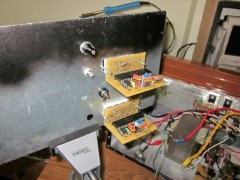Close# Digital LED voltmeter

Digital LED voltmeter

tnx to the publisher

You can see here a digital meter using the very popular IC 7107. IC2 is using for producing symmetrical supply(+/-5v) to the IC.

We can skip using 555 if we have a symmetrical sypply +/-5v.Figure shows a scheme of the voltmeter on the ice display, power supply circuit is +5 V.The negative voltage, which is necessary for proper operation, is generated by means of the IC2, C6, D1, D2 and C7. Circuit for generating a negative voltage according to the principle of operation is the same as proposed in the Data Sheet-u, in this case, instead of the integrated circuit 4009 then used NE555 timer, or in short 555, is used, it is because of this because it is smaller than said 4009th Instead of 4009 can also be used 4010, 4049 or 4050th 555 is used as a bound Schmitt Trigger, his ability to 200mA output current, which is more than capable 5-door with a buffer outputs from the 4009th Negative voltage is somewhere in the borders between 2.5 V and 3.5 V.

R1 =8K2
R2 = 47K / 470K
R3 = 100K
R4 = 2K
R5, R6 = 47K
R7 = 0R / 4K7
R8 = 560R
C1, C5, C6, C8, C9 = 100n
C2 = 470n / 47N
C3 = 220n
C4 = 100p
C7 = 10-22U
D1, D2 = 1N4148
IC1 = ICL7107
IC2 = NE555

Values of the elements apply to the area 200mV and 2V range, the values are different only for R2, C2 and R7. For 200mV range values are written in the first msetu, or the same as a typical scheme. For the 2V range values are written elsewhere. For R7 is that the area is used instead of 200mV inserted jumper wire. Displays which are used as a common anode, and having 10 lines, are used to SA52-11GWA or similar color as desired.

It is clear that there are not only two measuring zones, but it is possible to extend the measurement area with the corresponding series resistor at the entrance to the voltmeter. I recommend that the full scale of 200mV only be used for the voltmeter and ammeter for all other applications prefer to use the 2V range, and this has to be extended to area 20V or 200V range.

The display is 3 1/2 digit, which means that the maximum display 1999 course with a decimal point included if desired. Decimal point is connected through a resistor R8 to ground. It is also not necessary to use four displays, you can only use 3 to display 999 or range 100mV, 1V, 10V, 100V.

Input connector and HI and IN LO may not be connected to a voltage that is outside the power of ICL7107. The circuit is different from the typical applications even after the inlet side, as they are at the entrance of two resistors to protect the port from destruction in the event of over-voltage connections.This site uses Akismet to reduce spam. Learn how your comment data is processed.

© 2021 Electronics | WordPress Theme: Annina Free by CrestaProject.